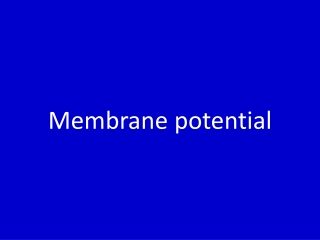DownloadDownload PresentationMembrane potential

# Membrane potential

Download Presentation## Membrane potential

- - - - - - - - - - - - - - - - - - - - - - - - - - - E N D - - - - - - - - - - - - - - - - - - - - - - - - - - -
##### Presentation Transcript

1. Membrane potential

2. Resting membrane potential • Action potential • End plate potential • Synaptic potential Excitatory post synaptic potential Inhibitatory post synaptic potential

3. Resting membrane potentialpotential

4. Resting Membrane Potential

5. GENESIS OF RESTING MEMBRANE POTENTIAL • PERMEABILITY OF Na +and K+ TO THE CELL MEMBRANE • Na +and K+ PUMP • NERNST POTENTIAL • Gibbs Donnan equilibrium potential • GOLDSMAN CONSTANT FIELD EQUATION

6. Cl- Na+ + + + + + + + + + + + + + + K+ + + + + + + + + - - - - - - - - - - - - - - - - - - - - - - K+ Cl- Na+ Resting Membrane Potential outside Membrane A- inside

7. K+ Na+ Cl- Outside of Cell Cell Membrane at rest Na+ - 70 mv A- K+ Cl- Inside of Cell Potassium (K+) can pass through to equalize its concentration Sodium and Chloride cannot pass through Result - inside is negative relative to outside The Cell Membrane is Semi-Permeable

8. Resting Membrane Potential (RMP) is the voltage (charge) difference across the cell membrane when the cell is at rest.  • RMP is a product of the distribution of charged particles (ions). • The membrane potential is always negative inside the cell, and varies in size from –20 to –200 mV in different cells and species (in humans it is –70mV).

9. This imbalance of ions causes a potential difference (or voltage) between the inside of the neurone and its surroundings, called the resting membrane potential. – + + 0 mV + + + – – -80 mV + – + – – + – + – – + – + – + + Voltmeter –

10. HOW DOES THE Na+/K+ PUMP AFFECT RMP? • The Na+/K+ Pump creates a concentration gradient by moving 3 Na+ out of the cell and 2 K+ into the cell. • The combination of the Na+/K+ATPase pump and the leak channels cause a stable imbalance of Na+ and K+ ions across the membrane.

11. Membrane Potential is always negative (-70mV) • K+ pass easily into the cell • Cl- and Na+ are impermeable • Negatively charged protein molecules inside the cell cannot pass the membrane

12. Nernst equation • Eqion= RT log ion i ZF ion o R- Gas constant T-temperature Z- valency of the ion F-Faraday constant

13. The membrane potential at which the electric force is equal in magnitude but opposite in direction to the concentration force is called equilibrium potential for that ion.

14. The equilibrium potential of a particular ion is usually designated by the notation Eion. • The equilibrium potential for any ion can be calculated using the Nernst equation . • EMF(millivolts) = ± 61log Conc inside/Conc outside • Equilibrium potential for Sodium (ENa) = +61 mV • Equilibrium potential for potassium(EK) = -90mV • Equilibrium potential for chloride(Ecl)= -70 mV

15. Nernst potential

16. Membrane Potential Caused by Diffusion of K+

17. Simplest Case Scenario: If a membrane were permeable to only K+ then… inside outside K+ K+ The electrical potential that counters net diffusion of K+ is called the K+ equilibrium potential (EK).

18. Membrane Potential Caused by Diffusion of Na+

19. Simplest Case Scenario: If a membrane were permeable to only Na+ then… inside outside Na+ Na+ The electrical potential that counters net diffusion of Na+ is called the Na+ equilibrium potential (ENa).

20. Vm -90 to -70 ENa +61 EK -94 Resting Membrane Potential 0 mV Why is Vm so close to EK? Ans. The membrane is far more permeable to K than Na..

21. Gibbs Donnan potential

22. Caused due to presence of impermeable anions K+ K+ Cl- Cl- Pr- At Equilibrium K+ K+ Cl- Cl- Pr-

23. At equilibrium • Diffusible cation concentration on the side containing pr- is greater than the other side. • Diffusible anion will be greater on the side without the non–diffusible anion. • This is known as Gibbs Donnan membrane equilibrium

24. Donnan equation K +in = cl-out K +out cl-in

25. Gibbs donnan effect • Because of excess protein on one side leads to increase in osmotically active particles-leads to rupture of cells –prevented by Na-K pump.

26. Goldman constant field equation • Vm = RT ln Pk K o +PNa Nao+Pcl Cli F PkK i + PNa Na i+Pcl Cl o